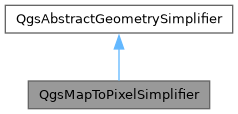QGIS API Documentation 3.33.0-Master (4676af859f)
Searching...
No Matches
QgsMapToPixelSimplifier Class Reference

Implementation of GeometrySimplifier using the "MapToPixel" algorithm. More...

`#include <qgsmaptopixelgeometrysimplifier.h>`

Inheritance diagram for QgsMapToPixelSimplifier:[legend]

## Public Types

enum  SimplifyAlgorithm { Distance = 0 , SnapToGrid = 1 , Visvalingam = 2 , SnappedToGridGlobal = 3 }
Types of simplification algorithms that can be used. More...

enum  SimplifyFlag { NoFlags = 0 , SimplifyGeometry = 1 , SimplifyEnvelope = 2 }
Applicable simplification flags. More...

## Public Member Functions

QgsMapToPixelSimplifier (int simplifyFlags, double tolerance, SimplifyAlgorithm simplifyAlgorithm=Distance)
Constructor.

bool isGeneralizableByMapBoundingBox (const QgsRectangle &envelope) const
Returns whether the envelope can be replaced by its BBOX when is applied the specified map2pixel context.

void setSimplifyAlgorithm (SimplifyAlgorithm simplifyAlgorithm)
Sets the local simplification algorithm of the vector layer managed.

void setSimplifyFlags (int simplifyFlags)
Sets the simplification hints of the vector layer managed.

void setTolerance (double value)
Sets the tolerance of the vector layer managed.

QgsAbstractGeometrysimplify (const QgsAbstractGeometry *geometry) const override
Returns a simplified version the specified geometry.

QgsGeometry simplify (const QgsGeometry &geometry) const override
Returns a simplified version the specified geometry.

SimplifyAlgorithm simplifyAlgorithm () const
Gets the local simplification algorithm of the vector layer managed.

int simplifyFlags () const
Gets the simplification hints of the vector layer managed.Public Member Functions inherited from QgsAbstractGeometrySimplifier
virtual ~QgsAbstractGeometrySimplifier ()=default

## Static Public Member Functions

static bool isGeneralizableByMapBoundingBox (const QgsRectangle &envelope, double map2pixelTol)
Returns whether the envelope can be replaced by its BBOX when is applied the specified map2pixel context.Static Public Member Functions inherited from QgsAbstractGeometrySimplifier
static bool isGeneralizableByDeviceBoundingBox (const QgsRectangle &envelope, float mapToPixelTol=1.0f)
Returns whether the device-envelope can be replaced by its BBOX when is applied the specified tolerance.

static bool isGeneralizableByDeviceBoundingBox (const QVector< QPointF > &points, float mapToPixelTol=1.0f)
Returns whether the device-geometry can be replaced by its BBOX when is applied the specified tolerance.

## Static Protected Member Functions

static float calculateLengthSquared2D (double x1, double y1, double x2, double y2)
Returns the squared 2D-distance of the vector defined by the two points specified.

static bool equalSnapToGrid (double x1, double y1, double x2, double y2, double gridOriginX, double gridOriginY, float gridInverseSizeXY)
Returns whether the points belong to the same grid.

## Protected Attributes

SimplifyAlgorithm mSimplifyAlgorithm
Current algorithm.

int mSimplifyFlags
Current simplification flags.

double mTolerance
Distance tolerance for the simplification.

## Detailed Description

Implementation of GeometrySimplifier using the "MapToPixel" algorithm.

Simplifies a geometry removing points within of the maximum distance difference that defines the MapToPixel info of a RenderContext request. This class enables simplify the geometries to be rendered in a MapCanvas target to speed up the vector drawing.

Definition at line 38 of file qgsmaptopixelgeometrysimplifier.h.

## ◆ SimplifyAlgorithm

Types of simplification algorithms that can be used.

Enumerator
Distance

The simplification uses the distance between points to remove duplicate points.

SnapToGrid

The simplification uses a grid (similar to ST_SnapToGrid) to remove duplicate points.

Visvalingam

The simplification gives each point in a line an importance weighting, so that least important points are removed first.

SnappedToGridGlobal

Snap to a global grid based on the tolerance. Good for consistent results for incoming vertices, regardless of their feature.

Definition at line 42 of file qgsmaptopixelgeometrysimplifier.h.

## ◆ SimplifyFlag

Applicable simplification flags.

Enumerator
NoFlags

No simplification can be applied.

SimplifyGeometry

The geometries can be simplified using the current map2pixel context state.

SimplifyEnvelope

The geometries can be fully simplified by its BoundingBox.

Definition at line 54 of file qgsmaptopixelgeometrysimplifier.h.

## ◆ QgsMapToPixelSimplifier()

 QgsMapToPixelSimplifier::QgsMapToPixelSimplifier ( int simplifyFlags, double tolerance, SimplifyAlgorithm simplifyAlgorithm = `Distance` )

Constructor.

Definition at line 31 of file qgsmaptopixelgeometrysimplifier.cpp.

## ◆ calculateLengthSquared2D()

 float QgsMapToPixelSimplifier::calculateLengthSquared2D ( double x1, double y1, double x2, double y2 )
staticprotected

Returns the squared 2D-distance of the vector defined by the two points specified.

Definition at line 41 of file qgsmaptopixelgeometrysimplifier.cpp.

## ◆ equalSnapToGrid()

 bool QgsMapToPixelSimplifier::equalSnapToGrid ( double x1, double y1, double x2, double y2, double gridOriginX, double gridOriginY, float gridInverseSizeXY )
staticprotected

Returns whether the points belong to the same grid.

Definition at line 49 of file qgsmaptopixelgeometrysimplifier.cpp.

## ◆ isGeneralizableByMapBoundingBox() [1/2]

 bool QgsMapToPixelSimplifier::isGeneralizableByMapBoundingBox ( const QgsRectangle & envelope ) const
inline

Returns whether the envelope can be replaced by its BBOX when is applied the specified map2pixel context.

Definition at line 105 of file qgsmaptopixelgeometrysimplifier.h.

## ◆ isGeneralizableByMapBoundingBox() [2/2]

 bool QgsMapToPixelSimplifier::isGeneralizableByMapBoundingBox ( const QgsRectangle & envelope, double map2pixelTol )
static

Returns whether the envelope can be replaced by its BBOX when is applied the specified map2pixel context.

Definition at line 412 of file qgsmaptopixelgeometrysimplifier.cpp.

## ◆ setSimplifyAlgorithm()

 void QgsMapToPixelSimplifier::setSimplifyAlgorithm ( SimplifyAlgorithm simplifyAlgorithm )
inline

Sets the local simplification algorithm of the vector layer managed.

Definition at line 90 of file qgsmaptopixelgeometrysimplifier.h.

## ◆ setSimplifyFlags()

 void QgsMapToPixelSimplifier::setSimplifyFlags ( int simplifyFlags )
inline

Sets the simplification hints of the vector layer managed.

Definition at line 85 of file qgsmaptopixelgeometrysimplifier.h.

## ◆ setTolerance()

 void QgsMapToPixelSimplifier::setTolerance ( double value )
inline

Sets the tolerance of the vector layer managed.

Definition at line 96 of file qgsmaptopixelgeometrysimplifier.h.

## ◆ simplify() [1/2]

 QgsAbstractGeometry * QgsMapToPixelSimplifier::simplify ( const QgsAbstractGeometry * geometry ) const
overridevirtual

Returns a simplified version the specified geometry.

Will return `nullptr` if no simplification is to be performed to the geometry.

Caller takes ownership of the returned geometry.

Since
QGIS 3.18

Implements QgsAbstractGeometrySimplifier.

Definition at line 456 of file qgsmaptopixelgeometrysimplifier.cpp.

## ◆ simplify() [2/2]

 QgsGeometry QgsMapToPixelSimplifier::simplify ( const QgsGeometry & geometry ) const
overridevirtual

Returns a simplified version the specified geometry.

Implements QgsAbstractGeometrySimplifier.

Definition at line 418 of file qgsmaptopixelgeometrysimplifier.cpp.

## ◆ simplifyAlgorithm()

 SimplifyAlgorithm QgsMapToPixelSimplifier::simplifyAlgorithm ( ) const
inline

Gets the local simplification algorithm of the vector layer managed.

Definition at line 88 of file qgsmaptopixelgeometrysimplifier.h.

## ◆ simplifyFlags()

 int QgsMapToPixelSimplifier::simplifyFlags ( ) const
inline

Gets the simplification hints of the vector layer managed.

Definition at line 83 of file qgsmaptopixelgeometrysimplifier.h.

## ◆ mSimplifyAlgorithm

 SimplifyAlgorithm QgsMapToPixelSimplifier::mSimplifyAlgorithm
protected

Current algorithm.

Definition at line 70 of file qgsmaptopixelgeometrysimplifier.h.

## ◆ mSimplifyFlags

 int QgsMapToPixelSimplifier::mSimplifyFlags
protected

Current simplification flags.

Definition at line 67 of file qgsmaptopixelgeometrysimplifier.h.

## ◆ mTolerance

 double QgsMapToPixelSimplifier::mTolerance
protected

Distance tolerance for the simplification.

Definition at line 73 of file qgsmaptopixelgeometrysimplifier.h.

The documentation for this class was generated from the following files: